September 30, 2023

# Simplification tricks and Questions for All Competitive Exams

Simplification  questions are  the most frequently asked questions in quantitative aptitude section for various Government exams. Candidates those who are preparing for various competitive exams must have to know the simple tricks to solve the simplification questions.

# What is simplification

A combination of numbers connected by one or more of the symbols  +, -, x,÷  and of is called a numerical expression . Thus  (i) 15 +8÷ 4-6   (ii) 16-43+8÷ 2 are examples of numerical expression.  Brackets are used to separate various parts of an expression. There are four kinds of brackets:

(i) Bar or Vinculum

(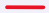)

(ii) Round brackets or small brackets    (     )

(iii) Curly brackets or braces        {    }

(iv) Square brackets or Big brackets          [      ]

The order of working with brackets is given below.

(i)Bar   (ii)  Small brackets    (iii) Curly brackets     (iv)   Big brackets

The rule to simplify an expression is ‘BODMAS‘  means

B -brackets       O- Order(powers/indices or roots)       D-Division         M= Multiplication       A-Addition

S-Subtraction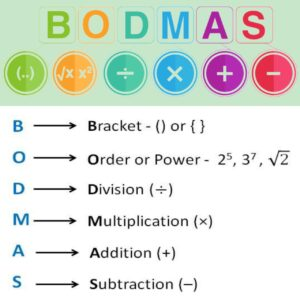Note : In the absence of any sign before a bracket  , we take the sign as multiplication.

## Simplification tips and tricks

No matter which  exam you are preparing for, simplification is one topic that can really help you to improve your  marks. Given below are some of the tips and tricks  by which you can  solve Simplification problems easily.

•  Learn the concept of BODMAS   and practice .
• Memorize tables upto 30 and learn  the different divisibility rules as it will help you to increase your speed.
• Memorize cubes  and squares of numbers up to 30.
• Learn some basic algebraic identities.  some of them are given below .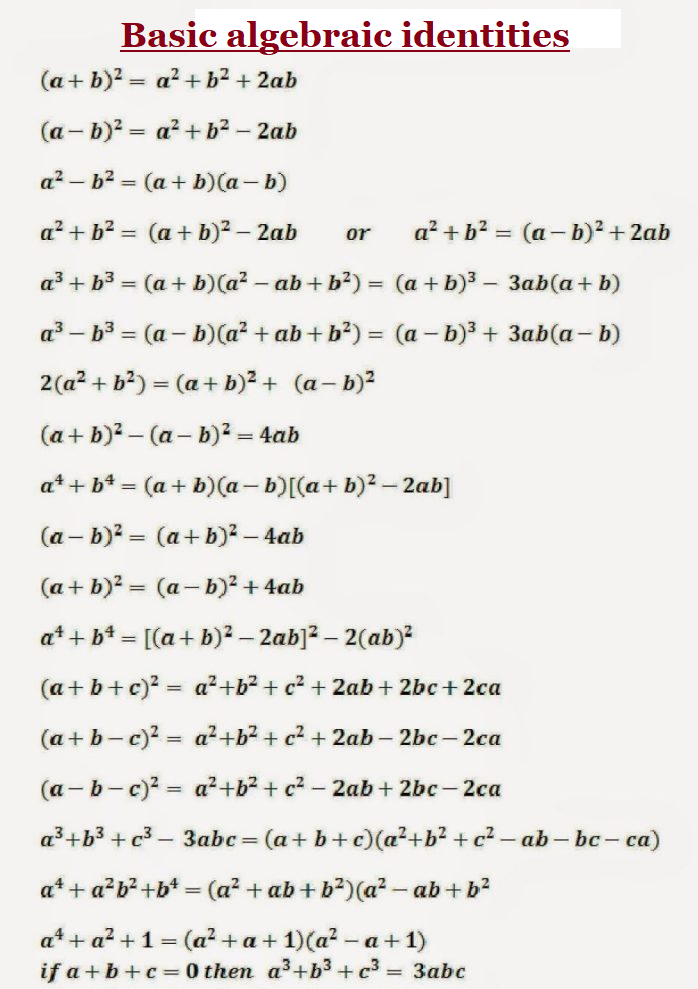• Learn the concept of percentages (conversion of fractions to percentage & percentage to fractions) .

### Simplification question (Solved examples)

BODMAS Simplification question

(i) Simplify  36  [18  {14  (15  4 ÷ 2 × 2)}]

Sol.  Given Expression

36  [18  {14  (15  4 ÷ 2 × 2)}]

= 36-[18-{14-(15-2  x 2 )}]               (solving division)

= 36-[18-{14-(15-4)}}                     (performing multiplication)

=36-[18-{14-11}}                            ( removing small brackets)

=36-[18-3]                                        (removing curly braces)

=36-15                                               (removing square brackets)
= 21

(ii)  Solve      27-[18-{16-(5-  )}]

Given Expression   27-[18-{16-(5-  )}]

=27-[18-{16-(5-3)}]                     (removing bar)

=27-[18-{16-2}]                            (removing  small brackets)

=27-[18-14]                                  (removing curly braces)

=27-4                                            ((removing square brackets)

=23

Fraction simplification

(i) Solve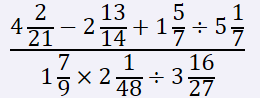Sol.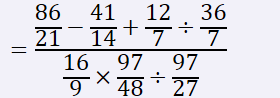=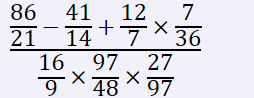=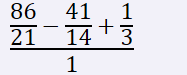=

(ii)

Sol.

=

=5997

(iii) If    then

Sol.

. Thus

Decimal Simplification

(i) Solve

Sol.

(using )

Simplification based on algebraic identities

(i) Solve

Sol.

=

=

Now using

=47+35+26= 108

(ii)

Sol.  =

( and )

=

=2

(iii)

Sol.

(   )

=888-222

=666

Surds Simplification

(i)  Solve

Sol.

=

=

=

=

BODMAS Questions PDF

You might also be interested in:

https://youtu.be/_LuPwBW4U4Q

https://youtu.be/JC71aL1citg#### Bina singh

View all posts by Bina singh →
ssc chsl 2023 tier 1 cut off NIRF Rankings 2023 : Top 10 Engineering colleges in India CBSE Compartment Exam 2023 Application Form SSC CGL 2023 Notification: Important Dates UPSC Recruitment 2023